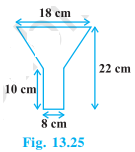# An oil funnel made of tin sheet consists of a 10 cm long cylindrical portion attached to a frustum of a cone. If the total height is 22 cm, diameter of the cylindrical portion is 8 cm and the diameter of the top of the funnel is 18 cm, find the area of the tin sheet required to make the funnel (see Fig.) .Given

Larger radius of frustum (R) = 18/2 = 9 cm

Smaller radius of frustum (r) = 8/2 = 4 cm

Height of frustum (H) = 22-10 = 12 cm

Radius of cylinder (r) = 8/2= 4 cm

Height of cylinder (h) = 10 cm

Solution

Area of the tin sheet required = CSA of frustum + CSA of cylinder

CSA of frustum = π(r+R)l

(l =√(H2 + (R-r)2))

l=√(122 + (9-4)2)

l = 13 cm

CSA of frustum = π(r+R)l

π×(9+4)×13

π×13×13

= 169π cm2

CSA of cylinder = 2πrh

2×π×4×10

= 80π cm2

Area of the tin sheet required = 169π + 80π

= 249π

Now let us consider π=22/7

= 782.571 cm2

the area of the tin sheet required to make the funnel is 782.571 cm2